# Mechanical Engineering - Online Test

Q1. Roots of the algebraic equation x3 + x2 + x + 1 = 0 are
Explaination / Solution:Q2. The threaded bolts A and B of same material and length are subjected to identical tensile load. If the elastic strain energy stored in bolt A is 4 times that of the bolt B and the mean diameter of bolt A is 12mm, the mean diameter of bolt B in mm is
Explaination / Solution: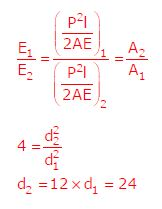Q3. For steady, fully developed flow inside a straight pipe of diameter D, neglecting gravity effects, the pressure drop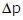over a length L and the wall shear stressare related by
Explaination / Solution: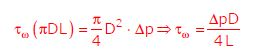Q4. Match the CORRECT pairs.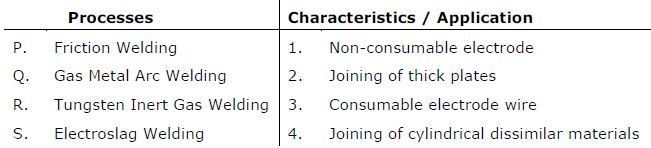Explaination / Solution:
No Explaination.

Q5. With K as a constant, the possible solution for the first order differential equationis
Explaination / Solution:Q6. The pressure, dry bulb temperature, and relative humidity of air in a room are1bar, 30ºC and 70% respectively. If the saturated steam pressure at 30ºC is 4.25kPa, the specific humidity of the room air in kg water vapour / kg dry air is
Explaination / Solution: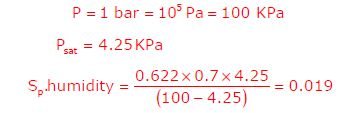Q7. In a rolling process, the state of stress of the material undergoing deformation is
Explaination / Solution:
No Explaination.

Q8. In simple exponential smoothing forecasting, to give higher weightage to recent demand information, the smoothing constant must be close to
Explaination / Solution:

Value of α close to one have less of a smoothing effect and give greater weight to recent changes in the data.

Q9. The function f(x) = 2x - x2 - x3 + 3  has
Explaination / Solution: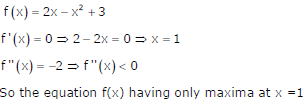Q10. A metric thread of pitch 2mm and thread angle 60º is inspected for its pitchdiameter using 3-wire method. The diameter of the best size wire in mm is
Explaination / Solution:

For 60º thread angle, best wire size =0.57135xP=1.154